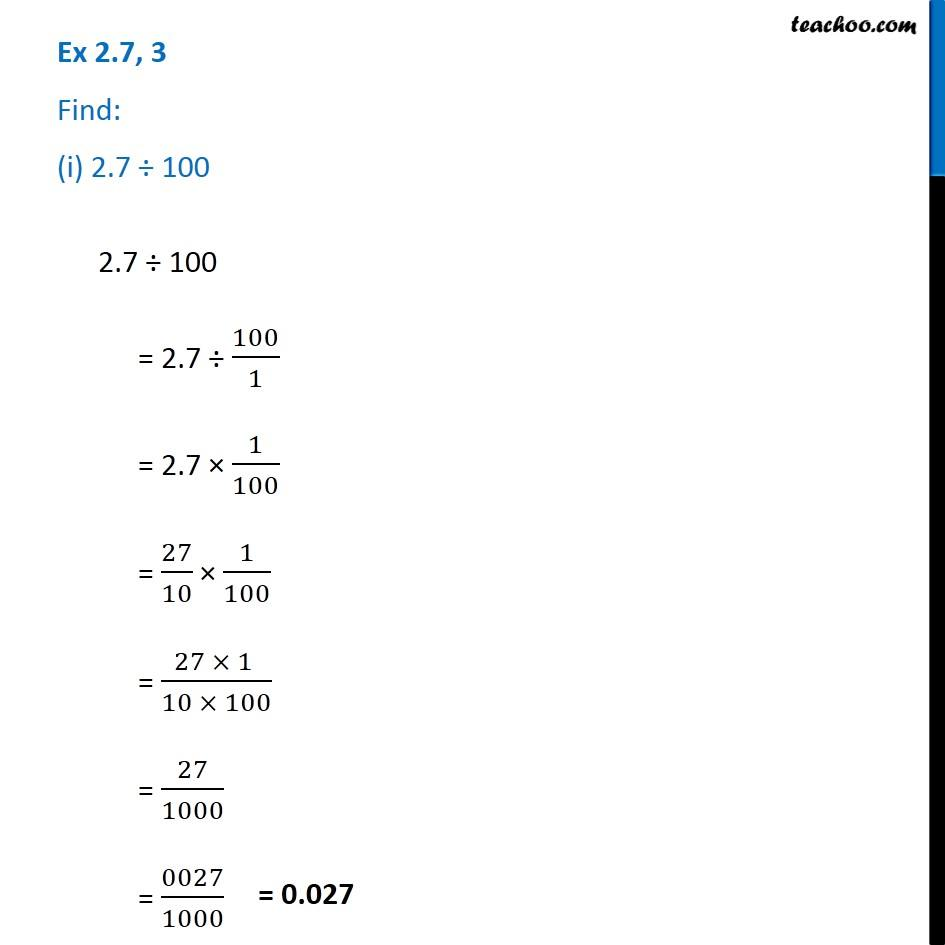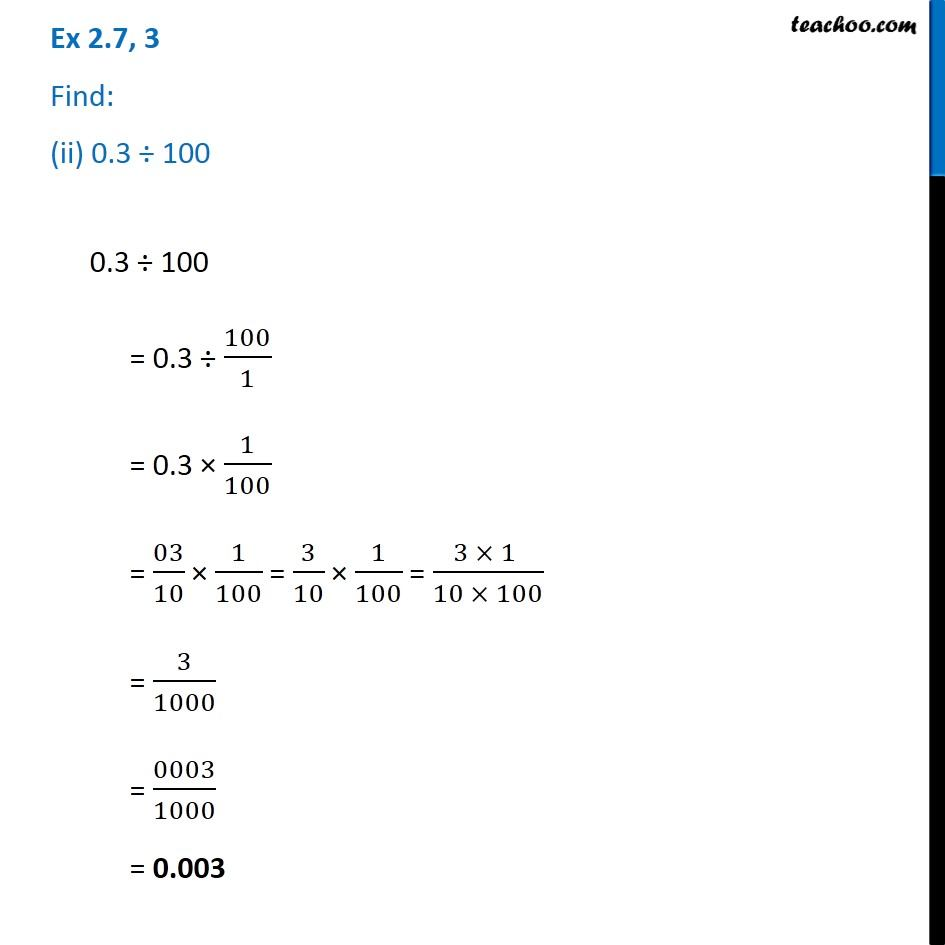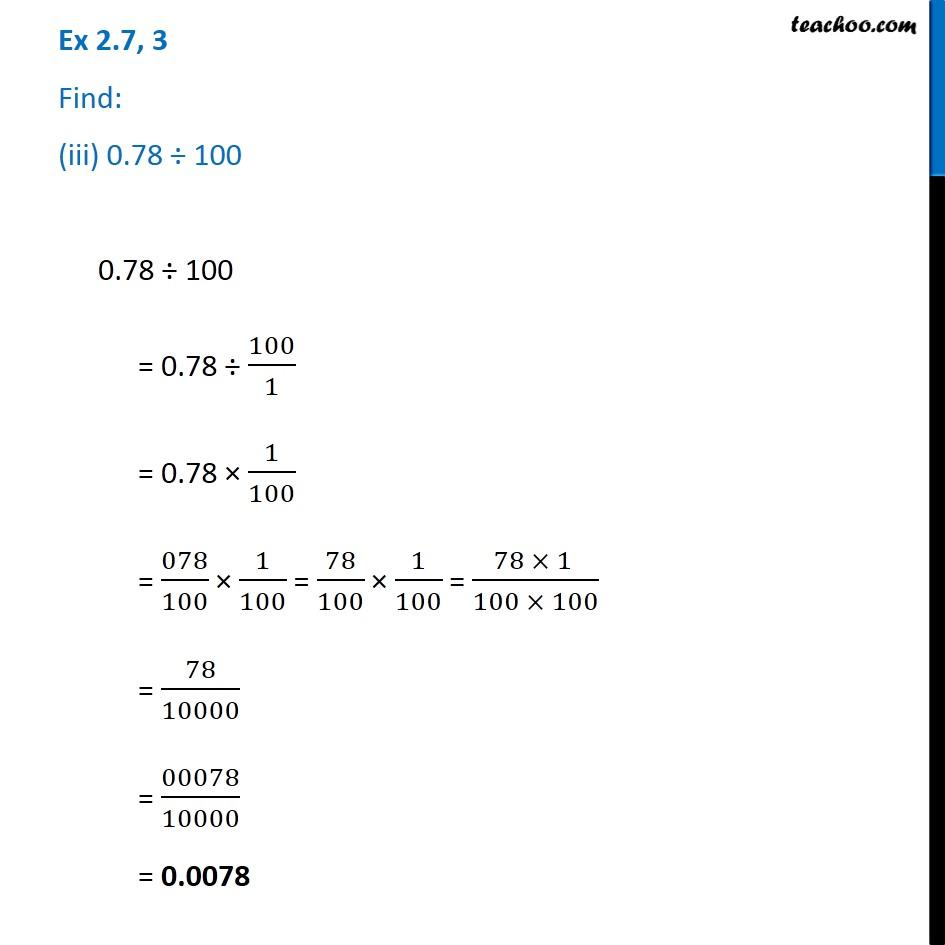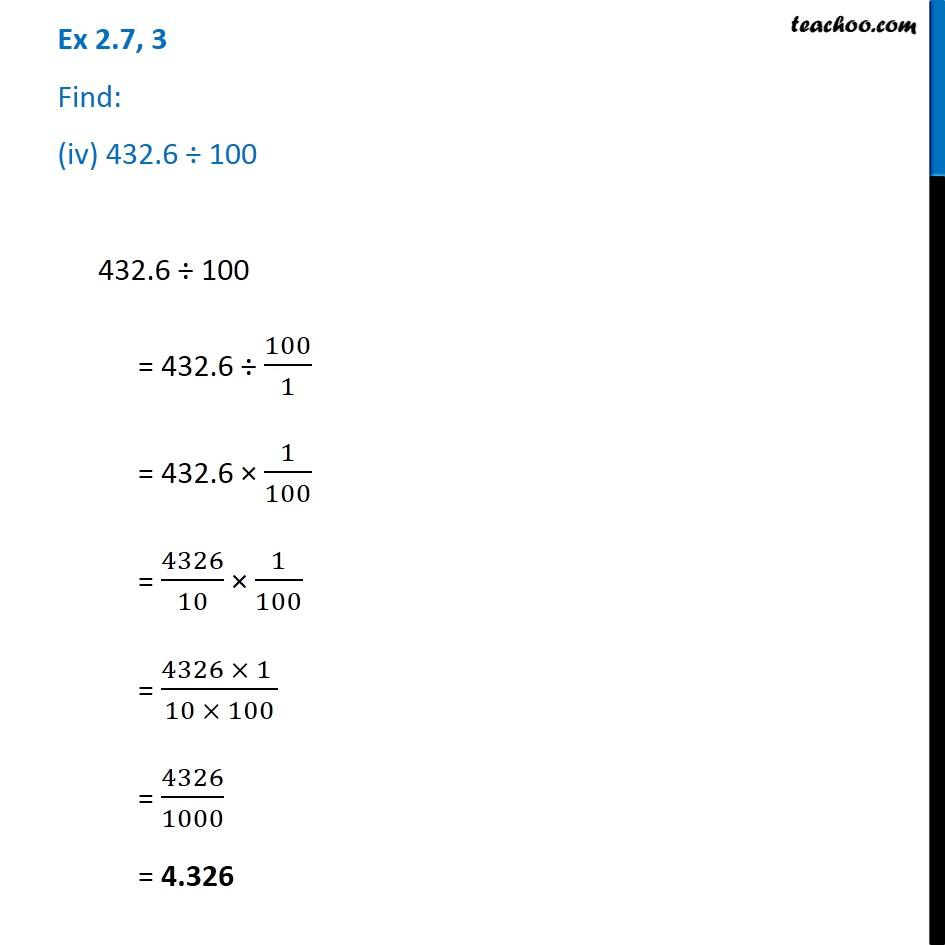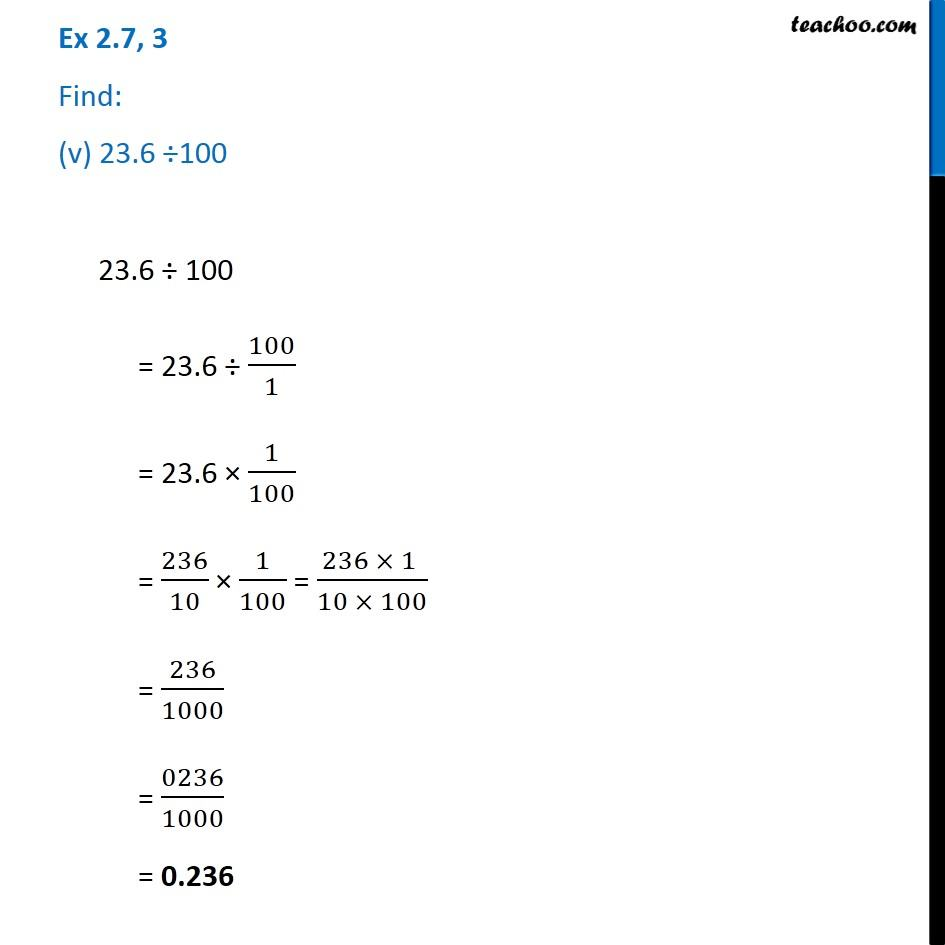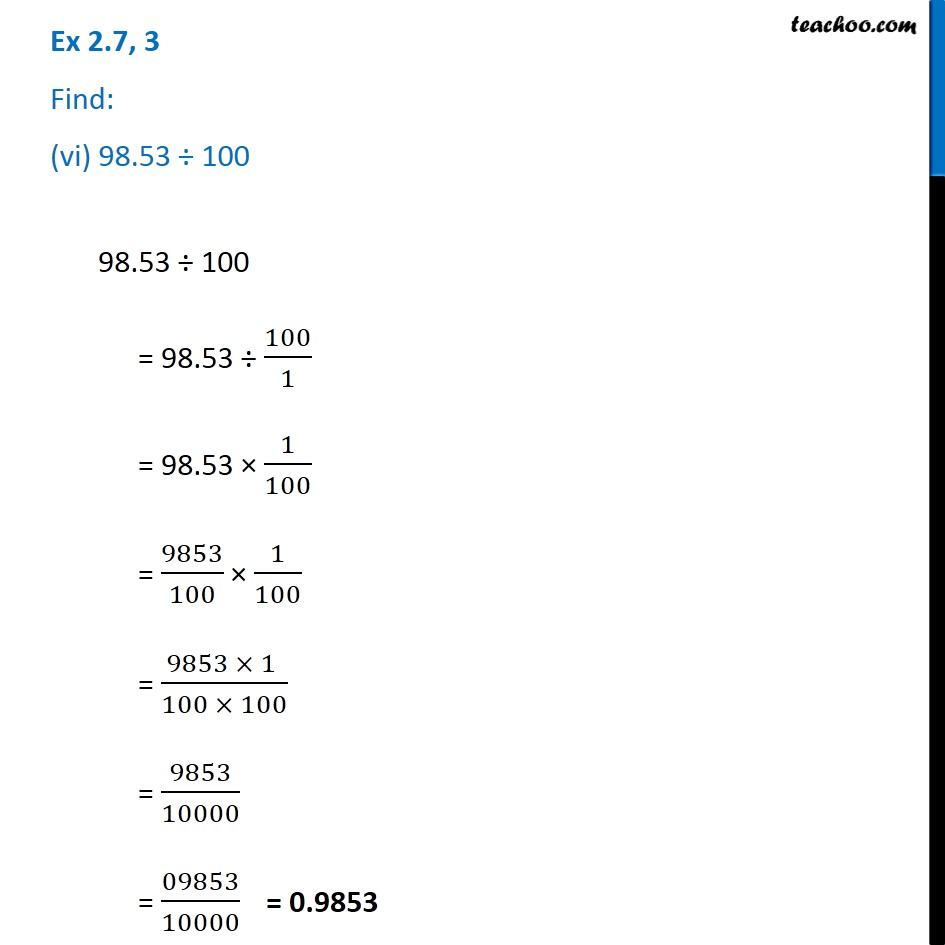Subscribe to our Youtube Channel - https://you.tube/teachoo

1. Chapter 2 Class 7 Fractions and Decimals
2. Concept wise
3. Division of Decimals

Transcript

Ex 2.7, 3 Find: (i) 2.7 ÷ 1002.7 ÷ 100 = 2.7 ÷ 100/1 = 2.7 × 1/100 = 27/10 × 1/100 = (27 × 1 )/(10 × 100) = 27/1000 = 0027/1000 Ex 2.7, 3 Find: (ii) 0.3 ÷ 1000.3 ÷ 100 = 0.3 ÷ 100/1 = 0.3 × 1/100 = 03/10 × 1/100 = 3/10 × 1/100 = (3 × 1 )/(10 × 100) = 3/1000 = 0003/1000 = 0.003 Ex 2.7, 3 Find: (iii) 0.78 ÷ 1000.78 ÷ 100 = 0.78 ÷ 100/1 = 0.78 × 1/100 = 078/100 × 1/100 = 78/100 × 1/100 = (78 × 1 )/(100 × 100) = 78/10000 = 00078/10000 = 0.0078 Ex 2.7, 3 Find: (iv) 432.6 ÷ 100432.6 ÷ 100 = 432.6 ÷ 100/1 = 432.6 × 1/100 = 4326/10 × 1/100 = (4326 × 1 )/(10 × 100) = 4326/1000 = 4.326

Division of Decimals